#2019 iT 邦幫忙鐵人賽DAY 7
2
AI & Data

## 在線資料中內插點

shapely的interpolate滿方便的，我們知道向量資料包含了點線面，線與面是由一序列的點組成的，shapely的interpolate提供了一個方法，可以在這樣的序列資料中，沿著序列的方向內插一個點。

``````import geopandas as gpd
from shapely.geometry import LineString,Point
line1 = LineString([(0, 0), (50, 50), (100, 100)])
line2 = LineString([(10, 0), (60, 50), (110, 100)])
lines = gpd.GeoDataFrame()
lines['geometry'] = [line1,line2]
lines.plot()
``````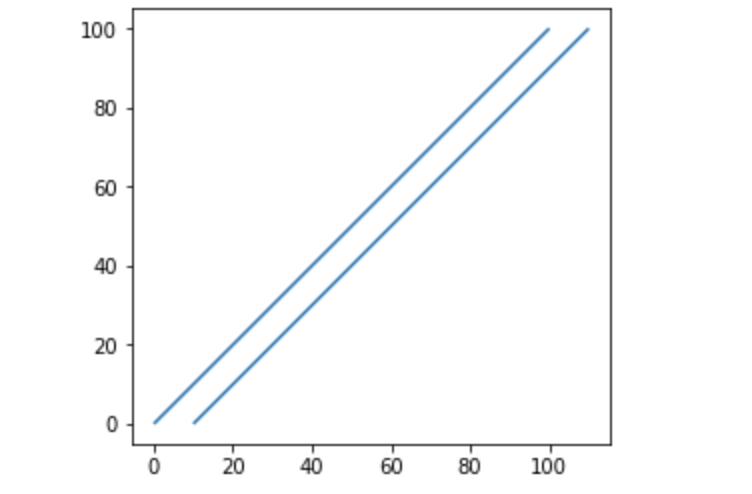``````base=lines.plot()
points = gpd.GeoDataFrame()
points['geometry'] = [row['geometry'].interpolate(50) for idx,row in df.iterrows()]
points.plot(ax=base)
``````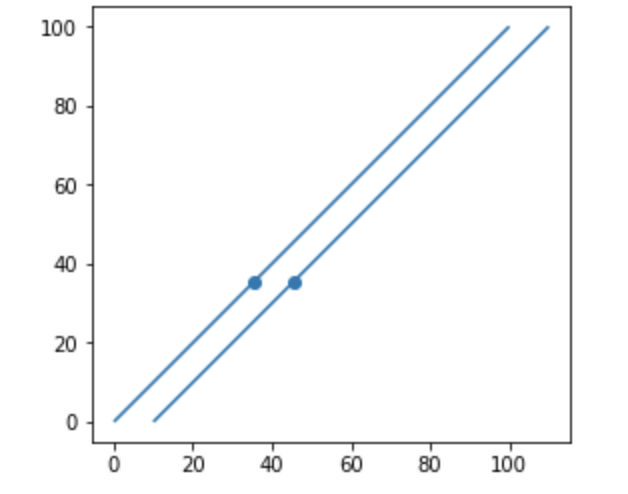``````base=lines.plot()
points = gpd.GeoDataFrame()
intpoints=[row['geometry'].interpolate(50) for idx,row in df.iterrows()]
intpoints+=[row['geometry'].interpolate(25) for idx,row in df.iterrows()]
intpoints+=[row['geometry'].interpolate(75) for idx,row in df.iterrows()]
points['geometry'] = intpoints
points.plot(ax=base)
``````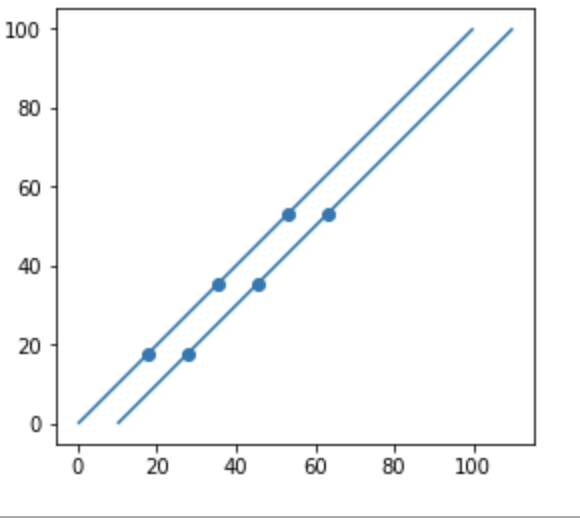## project

`shapely``project`這個方法的意思跟地圖投影無關，

``````LineString([(0, 0), (50, 50), (100, 100)]).project(Point(50,0))
``````

``````lines = gpd.GeoDataFrame()
lines['geometry'] = [LineString([(0, 0), (50, 50), (100, 100)])]
base=lines.plot()

points = gpd.GeoDataFrame()
points['geometry'] = [Point(50,0)]
points.plot(ax=base)
``````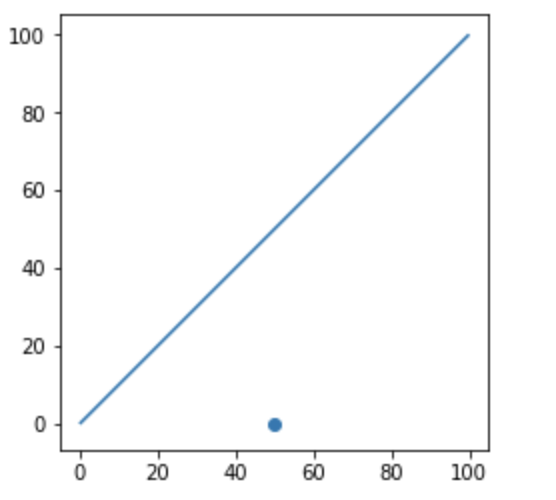project方法提供的，是(50,0)這個點，投影在線上的點p，這個點p距離線的原點(0,0)的距離

``````lines = gpd.GeoDataFrame()
lines['geometry'] = [LineString([(0, 0), (50, 50), (100, 100)])]
base=lines.plot()

points = gpd.GeoDataFrame()
points['geometry'] = [Point(50,50)]
points.plot(ax=base)
LineString([(0, 0), (50, 50), (100, 100)]).project(Point(50,50))
``````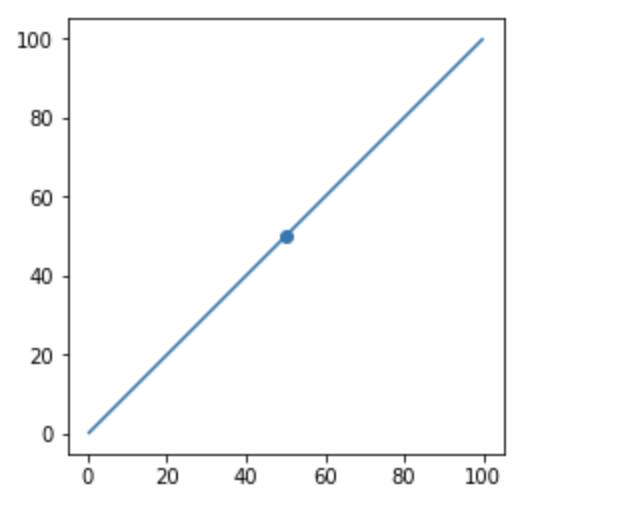## de-9im-relationships

de-9im全名是(Dimensionally Extended nine-Intersection Model)，是一個GIS位向關係描述的模型及標轉，這個模型描述了兩個幾何物件的關係，它把兩個幾何物件的關係以物件的I(Interior),B(Boundary),E(Exterior)，兩物件之間的幾何關係以此3*3內的關係來判斷，這個矩陣就是de-9im，以下圖為例：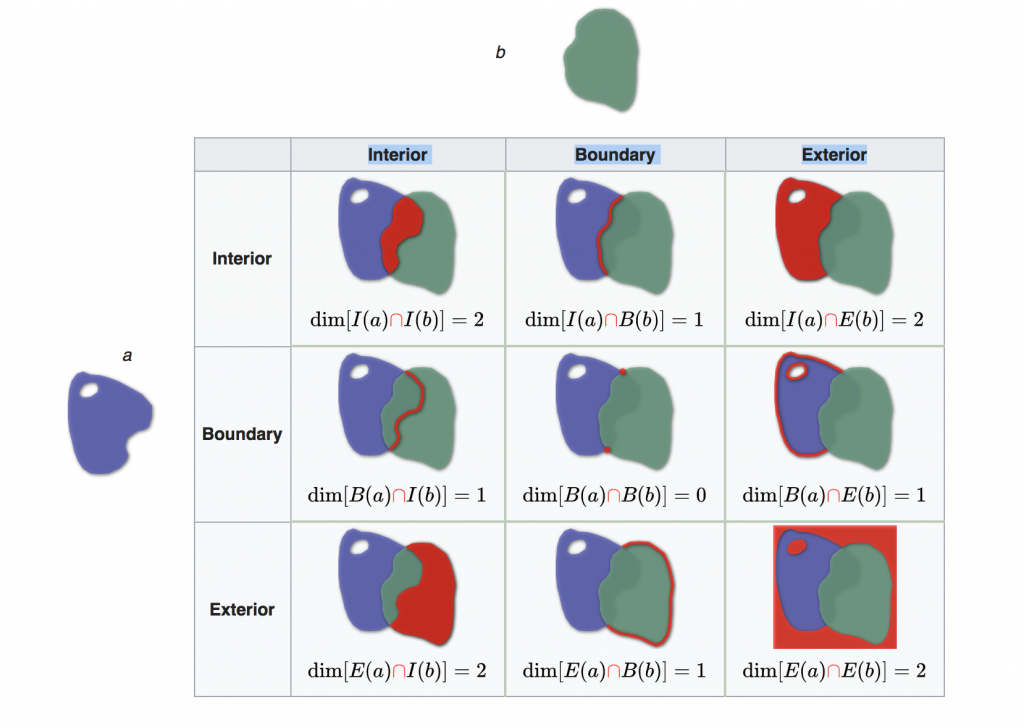[取自DE-9IM - Wikipedia]

`TTTTTTTTT`

`T********`

`FF*FF****`

``````p1=gpd.read_file('output/Library.shp',encoding='utf-8').head(1)
p1.crs = {'init' :'epsg:4326'} # 避免資料沒設，這邊再重新給一次
p1=p1.to_crs(epsg=3826)
p1['geometry']=p1.buffer(30).translate(xoff=20.0, yoff=0.0)

p2.crs = {'init' :'epsg:4326'} # 避免資料沒設，這邊再重新給一次
p2=p2.to_crs(epsg=3826)
p2['geometry']=p2.buffer(30)
base=p1.plot(color='blue')
p2.plot(ax=base,color='brown')
``````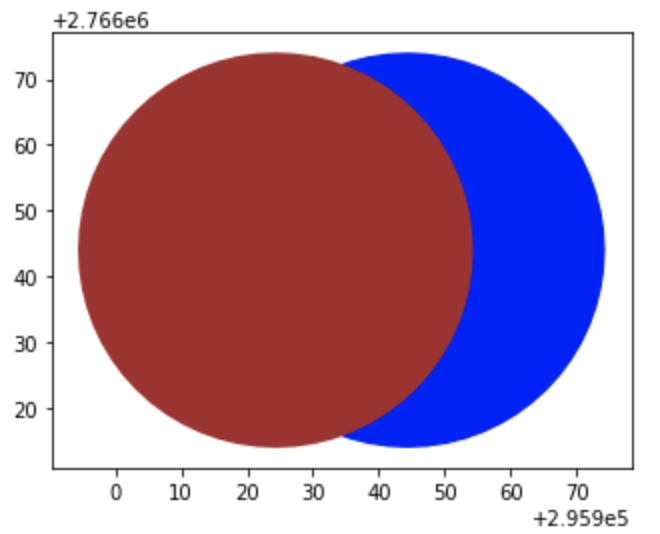``````p1.at[0,'geometry'].relate(p2.at[0,'geometry'])
``````